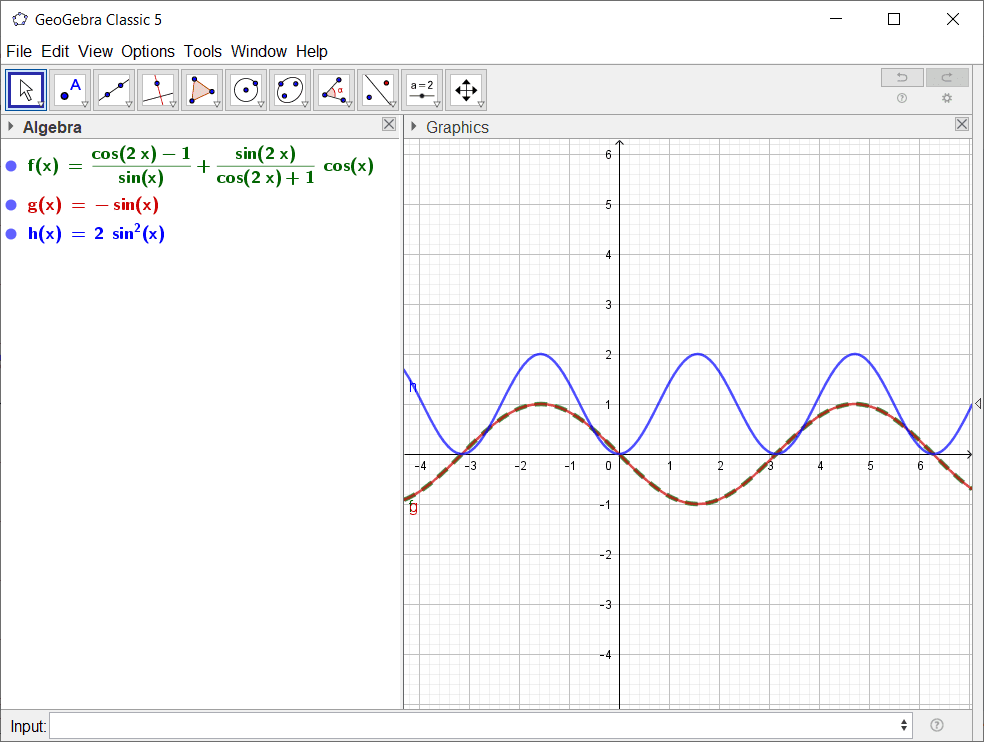# Trigonometry (re-moved to Precalc Math Homework)

Crystal037
Homework Statement: (cos2A -1)/sinA +sin2A/(cos2A+1)*cosA=__________________
Homework Equations: sin2A=2sinAcosA
cos2A+1=cos^2A
cos2A-1=sin^2A

(cos2A -1)/sinA +sin2A/(cos2A+1)*cosA
[cos^2(2A)-1 +2sin^2(A)cos^2(A)]/sinA(cos2A+1)
[-sin^2(2A) + 2sin^2(A)cos^2(A)]/sinA(cos2A+1)
I can't proceed any further.
The answer must be 2 sin^2A

Homework Helper
Gold Member
The second and third identities that you use are wrong.

Crystal037
cos2A+1=2cos^2A
cos2A-1=2sin^2A
Yeah I typed it wrong.
But I am still not getting the answer.
Give me a hint on how to proceed.
(cos2A -1)/sinA +sin2A/(cos2A+1)*cosA
[cos^2(2A)-1 +2sin^2(A)cos^2(A)]/sinA(cos2A+1)
[-sin^2(2A) + 2sin^2(A)cos^2(A)]/sinA(cos2A+1)

Homework Helper
Gold Member
Your second identity has the wrong sign. ##\cos(2A) -1 = -2\sin^2(A)##

Crystal037
[cos^2(2A)-1 +2sin^2(A)cos^2(A)]/sinA(cos2A+1)
=[cos^2(2A)-1 + sin^2(2A)/2]/sinA(cos2A+1)
=[cos^2(2A)-1]/2sinA(cos2A+1)
=[cos(2A)-1]/2sinA
=-2sin^2A/2sin(A)
=-sinA
But this isn't correct when I put some values of A for eg. 90 degrees and 45 degrees

•FactChecker
Homework Helper
Gold Member
That is the correct answer. I don't know where the proposed answer of ##2\sin^2(A)## in the original post came from.

Crystal037
The answer 2sin^2(A) was given as the answer in the book. But when I put any value of A, like 90 I don't get the LHS and RHS EQUAL. Also when I put A=90 in the answer I got I didn't get LHS = RHS

Homework Helper
Gold Member
Right. Something is wrong there. Those two are not identical.

Crystal037
Can you please tell me where's the mistake

Homework Helper
Gold Member
Can you please tell me where's the mistake
Make sure that you read the problem correctly. You got the right answer and it is not what you expected from your problem statement. Maybe the book is wrong.

Crystal037
What do you mean? Elaborate

Homework Helper
Gold MemberFunctions f and g are identical. Function h is not the same as function f.

•Crystal037
Crystal037
but f( 90 degrees )=-2 when solved through the LHS part, then why in the graph it is-1

Homework Helper
Gold Member
You tell me. Are you sure that you are handling the zero of the denominator ##(\cos(2A)+1)## correctly? Actually, the function f is not defined at 90 degrees, but it can be smoothly extended to include that point.

Crystal037
How come the L.H.S of an equation is not defined for a point but the RHS value is. Then both the functions are not equal and our equality shouldn't hold

Mentor
Consider e.g. ##\displaystyle \frac{x^2}{x} = x##. Is this equation always true? It looks so - but for x=0 the left hand side is undefined while the right hand side is 0. Everywhere else the equation is true, so the two sides are still "sort of" equal. Equal at every point where the left hand side is defined.

•Crystal037 and FactChecker
•